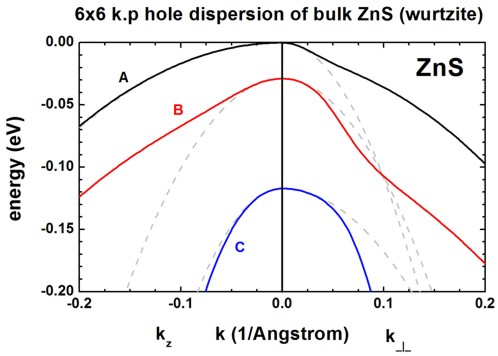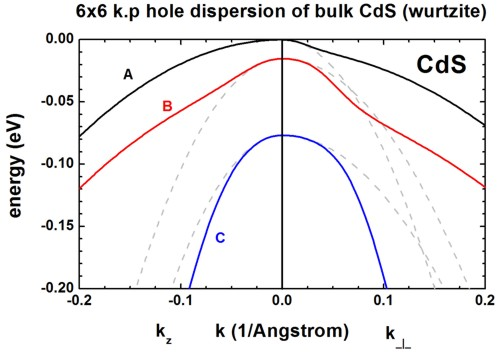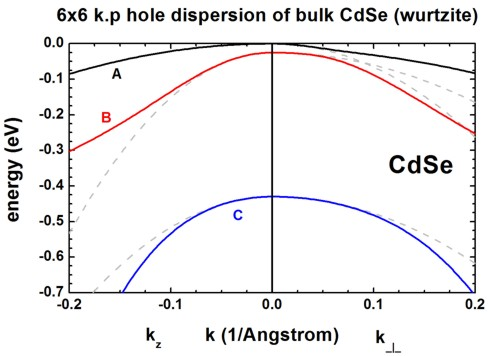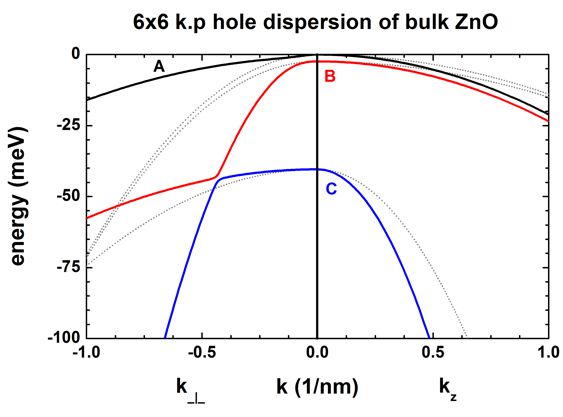nextnano.com  GUI: nextnanomat  Tool: nextnano++  Tool: nextnano³  Tool: nextnano.QCL  Download | Search | Copyright | News | Publications  * password protected nextnano³ software1D bulk k.p dispersion in II-VI

# nextnano3 - Tutorial

## k.p dispersion in bulk unstrained ZnS, CdS, CdSe and ZnO (wurtzite)

Author: Stefan Birner

If you want to obtain the input files that are used within this tutorial, please contact stefan.birner@nextnano.de.
```-> bulk_6x6kp_dispersion_ZnS_nn3.in -> bulk_6x6kp_dispersion_CdS_nn3.in -> bulk_6x6kp_dispersion_CdSe_nn3.in -> bulk_6x6kp_dispersion_ZnO_nn3.in  / *_nnp.in - ```input file for nextnano3 / nextnano++ software```  ```

## k.p dispersion in bulk unstrained ZnS, CdS, CdSe and ZnO (wurtzite)

This tutorial is based on

Valence band parameters of wurtzite materials
J.-B. Jeon, Yu.M. Sirenko, K.W. Kim, M.A. Littlejohn, M.A. Stroscio
Solid State Communications 99, 423 (1996)

• We want to calculate the dispersion E(k) from |k|=0 [1/nm] to |k|=2.0 [1/nm] along the following directions in k space:
-  to , i.e. parallel to the c axis (Note: The c axis is parallel to the z axis.)
-  to , i.e. perpendicular to the c axis (Note: The (x,y) plane is perpendicular to the c axis.)
We compare 6-band k.p theory results vs. single-band (effective-mass) results.
• We calculate E(k) for bulk ZnS, CdS and CdSe (unstrained).

### Bulk dispersion along  and 

• ``` \$output-kp-data  destination-directory  = kp/  bulk-kp-dispersion     = yes  grid-position          = 5d0              ! ```in units of` [nm]```` ``````  !-----------------------------------------------------------------------------------  ! Dispersion along  direction, i.e. parallel      to c= axis in wurtzite  ! Dispersion along  direction, i.e. perpendicular to c= axis in wurtzite  ! maximum |k| vector = 2.0 [1/nm]  !-----------------------------------------------------------------------------------  k-direction-from-k-point = 0d0          0d0          2.0d0 ! ``` k-direction and range for dispersion plot``` [1/nm]  k-direction-to-k-point   = 1.41421356d0 1.41421356d0 0d0   ! ```k-direction and range for dispersion plot``` [1/nm]  ! ```The dispersion is calculated from the k point '`k-direction-from-k-point`' to Gamma, and then from the Gamma point to '`k-direction-to-k-point`'.```  number-of-k-points       = 100             ! ```number of k points to be calculated (resolution)
` \$end_output-kp-data`
• We calculate the pure bulk dispersion at` grid-position=5d0`, i.e. for the material located at the grid point at 5 nm. In our case this is ZnS but it could be any strained alloy.
In the latter case, the k.p Bir-Pikus strain Hamiltonian will be diagonalized.
The grid point at` grid-position `must be located inside a quantum cluster.
`shift-holes-to-zero = yes `forces the top of the valence band to be located at 0 eV.
How often the bulk k.p Hamiltonian should be solved can be specified via` number-of-k-points`. To increase the resolution, just increase this number.
• The maximum value of |k| is 2.0 [1/nm].
Note that for values of |k| larger than 2.0 [1/nm], k.p theory might not be a good approximation any more.
This depends on the material system, of course.
• Start the calculation.
The results can be found in:```  kp_bulk/bulk_6x6kp_dispersion_as_in_inputfile_kxkykz_000_kxkykz.dat ``` (6-band k.p)
`  kp_bulk/bulk_sg_dispersion.dat                                      `(single-band approximation)

`bulk_6x6kp_dispersion_as_in_inputfile_kxkykz_000_kxkykz.dat`:
The first column contains the |k| vector in units of [1/nm], the next six columns the six eigenvalues of the 6-band k.p Hamiltonian for this k=(kx,ky,kz) point.
The resulting energy dispersion is usually discussed in terms of a nonparabolic and anisotropric energy dispersion of heavy, light and split-off holes, including valence band mixing.

`bulk_sg_dispersion.dat`:
The first column contains the |k| vector in units of [1/nm], the next three columns the energy for heavy (A), light (B) and crystal-field split-off (C) hole  for this k=(kx,ky,kz) point.
The single-band effective mass dispersion is parabolic and depends on a single parameter: The effective mass m*.
Note that in wurtzite materials, the mass tensor is usually anisotropic with a mass mzz parallel to the c axis, and two masses perpendicular to it mxx=myy.

### Results

• Here we visualize the results.
The final figures will look like this (left: dispersion along , right: dispersion along ):• These three figures are in excellent agreement to Figure 1 of the paper by [Jeon].
• The dispersion along the hexagonal c axis is substantially different than the dispersion in the plane perpendicular to the c axis.
The effective mass approximation is indicated by the dashed, grey lines.
For the heavy holes (A), the effective mass approximation is very good for the dispersion along the c axis, even at large k vectors.

• For comparison, the single-band (effective-mass) dispersion is also shown. For ZnS, it corresponds to the following effective hole masses:
```  valence-band-masses = 0.35d0  0.35d0  2.23d0 ! [m0] ```heavy hole A  (`2.23 `along c axis)```                       0.485d0 0.485d0 0.53d0 ! [m0] ```light    hole B  (`0.53 `along c axis)```                       0.75d0  0.75d0  0.32d0 ! [m0] ```crystal hole C  (`0.32 `along c axis)``` ```The effective mass approximation is a simple parabolic dispersion which is anisotropic if the mass tensor is anisotropic (i.e. it also depends on the k vector direction).

One can see that for |k| < 0.5 [1/nm] the single-band approximation is in excellent agreement with 6-band k.p but differs at larger |k| values substantially.

• Plotting E(k) in three dimensions
Alternatively one can print out the 3D data field of the bulk E(k) = E(kx,ky,kz) dispersion.

``` \$output-kp-data  ...  bulk-kp-dispersion-3D  = yes !----------------------------------------! maximum |k| vector = 2.0 [1/nm]!----------------------------------------  k-direction-to-k-point = 0d0  0d0 2.0d0   ! ```k-direction and range for dispersion plot``` [1/nm]  number-of-k-points     = 40               ! ```number of k points to calculated (resolution)

The meaning of``` number-of-k-points = 41``` is the following:
40 k points from '```- maximum |k| vector' ```to zero (plus the Gamma point) and
40 k points from zero to  '`+ maximum |k| vector'` (plus the Gamma point) along all three directions,
i.e. the whole 3D volume then contains 81 * 81 * 81 = 531441 k points.

## k.p dispersion in bulk unstrained ZnO

The following figure shows the bulk 6-band k.p energy dispersion for ZnO.
The gray lines are the dispersions assuming a parabolic effective mass.The following files are plotted:
```- kp_bulk/bulk_6x6kp_dispersion_as_in_inputfile_kxkykz_000_kxkykz.dat - kp_bulk/bulk_sg_dispersion.dat ```The files``` - bulk_6x6kp_dispersion_axis_-100_000_100.dat ```and
`- bulk_6x6kp_dispersion_diagonal_-110_000_1-10.dat`
contain the same data because for a wurtzite crystal, due to symmetry, the dispersion in the plane perpendicular to the kz direction (corresponding to ) is isotropic.

• Please help us to improve our tutorial! Send comments to``` support [at] nextnano.com```.